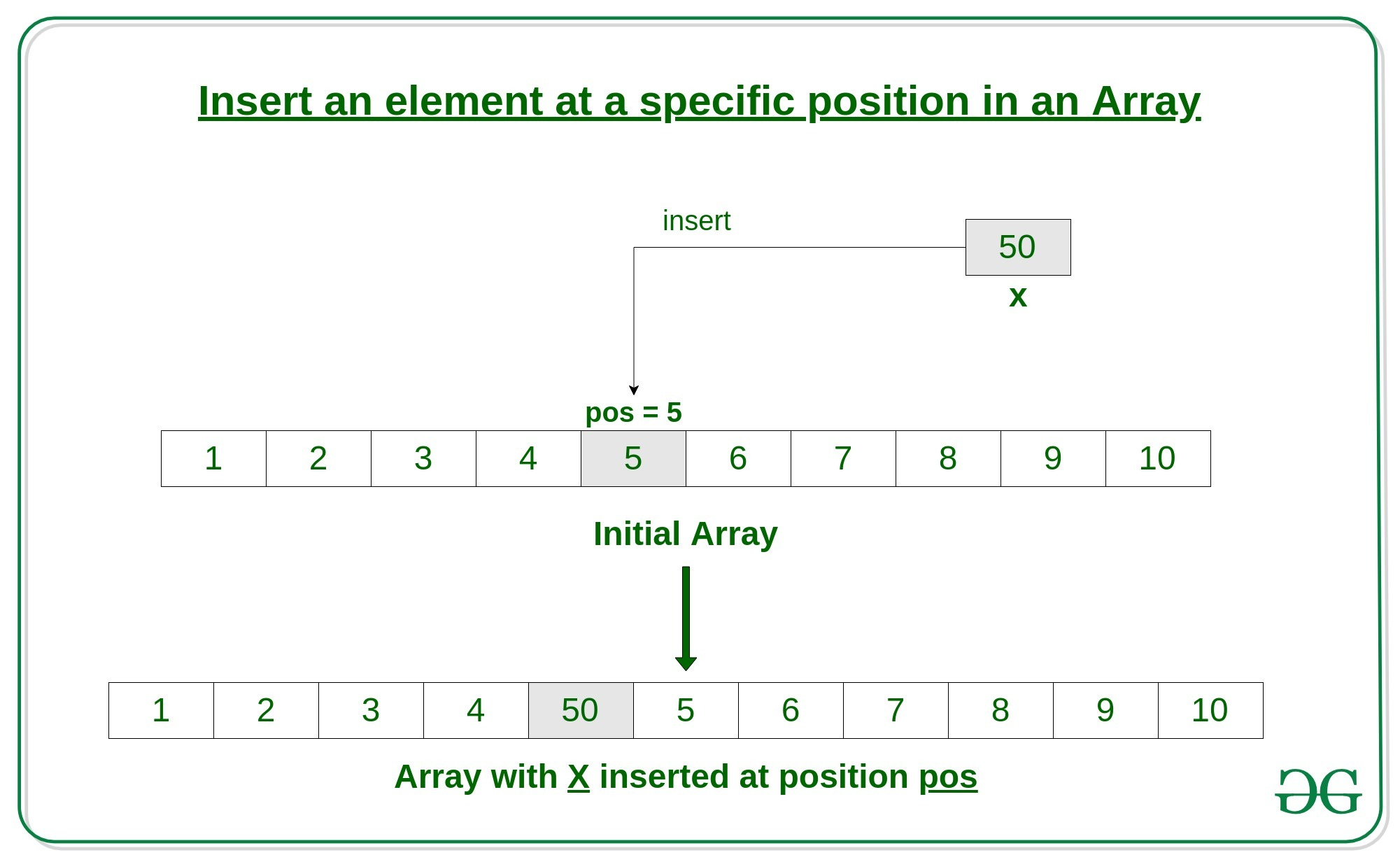# C program to Insert an element in an Array

An array is a collection of items stored at contiguous memory locations. In this article, we will see how to insert an element in an array in C.

Given an array arr of size n, this article tells how to insert an element x in this array arr at a specific position pos.Approach:
Here’s how to do it.

1. First get the element to be inserted, say x
2. Then get the position at which this element is to be inserted, say pos
3. Then shift the array elements from this position to one position forward, and do this for all the other elements next to pos.
4. Insert the element x now at the position pos, as this is now empty.

Below is the implementation of the above approach:

 `// C Program to Insert an element ` `// at a specific position in an Array ` ` `  `#include ` ` `  `int` `main() ` `{ ` `    ``int` `arr = { 0 }; ` `    ``int` `i, x, pos, n = 10; ` ` `  `    ``// initial array of size 10 ` `    ``for` `(i = 0; i < 10; i++) ` `        ``arr[i] = i + 1; ` ` `  `    ``// print the original array ` `    ``for` `(i = 0; i < n; i++) ` `        ``printf``(``"%d "``, arr[i]); ` `    ``printf``(``"\n"``); ` ` `  `    ``// element to be inserted ` `    ``x = 50; ` ` `  `    ``// position at which element ` `    ``// is to be inserted ` `    ``pos = 5; ` ` `  `    ``// increase the size by 1 ` `    ``n++; ` ` `  `    ``// shift elements forward ` `    ``for` `(i = n; i >= pos; i--) ` `        ``arr[i] = arr[i - 1]; ` ` `  `    ``// insert x at pos ` `    ``arr[pos - 1] = x; ` ` `  `    ``// print the updated array ` `    ``for` `(i = 0; i < n; i++) ` `        ``printf``(``"%d "``, arr[i]); ` `    ``printf``(``"\n"``); ` ` `  `    ``return` `0; ` `} `

Output:

```1 2 3 4 5 6 7 8 9 10
1 2 3 4 50 5 6 7 8 9 10
```

My Personal Notes arrow_drop_upCheck out this Author's contributed articles.

If you like GeeksforGeeks and would like to contribute, you can also write an article using contribute.geeksforgeeks.org or mail your article to contribute@geeksforgeeks.org. See your article appearing on the GeeksforGeeks main page and help other Geeks.

Please Improve this article if you find anything incorrect by clicking on the "Improve Article" button below.

Article Tags :
Practice Tags :

Be the First to upvote.

Please write to us at contribute@geeksforgeeks.org to report any issue with the above content.